Time Travel Research Center © 1998 Cetin BAL - GSM:+90  05366063183 -Turkey/Denizli  Quantum electrodynamics Quantum electrodynamics (QED) is a relativistic quantum field theory of electromagnetism. QED mathematically describes all phenomena involving electrically charged particles interacting by means of the electromagnetic force, whether the interaction is between light and matter or between two charged particles. It has been called "the jewel of physics" for its extremely accurate predictions of quantities like the anomalous magnetic moment of the muon, and the Lamb shift of the energy levels of hydrogen. Contents 1 Physical interpretation of QED 2 History 3 Mathematics 4 See also 5 References 6 External links Physical interpretation of QED In classical physics, light is observed to take the quickest path between two points; but how does light `know where it's going'? That is, if the start and end points are known, the path that will take the shortest time can be calculated. However, when light is first emitted, the end point is not known, so how is it that light always takes the quickest path? The answer is provided by QED. Light doesn't know where it is going, and it doesn't always take the quickest path. In fact, according to QED, light can go slower or faster than the speed of light to get there. Physically, QED describes charged particles (and their antiparticles) interacting with each other by the exchange of photons. The magnitude of these interactions can be computed using perturbation theory; these rather complex formulas have a remarkable pictorial representation as Feynman diagrams . QED was historically the theory to which Feynman diagrams were first applied. These diagrams were invented on the basis of Lagrangian mechanics. QED doesn't predict what will happen in an experiment. But it can predict the probability of what will happen in an experiment, which is how it is experimentally verified. Using a Feynman diagram one decides every possible path between the start and end points. Each path is assigned a complex number-valued probability and the actual path we observe is the weighted average of all of the paths. This average path is the one that the classical theory predicts, the quickest path between the two points. An exposition from this point of view of QED is provided in Feynman's classic: QED: The strange theory of light and matter (see below). History In 1900, Max Planck introduced the idea that energy is quantized in order to derive a formula that could predict the observed frequency dependence of the energy emitted by a black body. In 1905, Einstein explained the photoelectric effect by postulating that light energy comes in quanta called photons. In 1913, Bohr explained the spectral lines of the hydrogen atom, again by using quantization. In 1924, Louis de Broglie put forward his theory of matter waves. These theories, though successful, were strictly phenomenological: they provided no rigorous justification for the quantization they employed. They are collectively known as the old quantum theory. The phrase "quantum physics" was first used in Johnston's Planck's Universe in Light of Modern Physics. Modern quantum mechanics was born in 1925 when Werner Heisenberg developed matrix mechanics and Erwin Schrödinger invented wave mechanics and the Schrödinger equation. Schrödinger subsequently showed that these two approaches were equivalent. Heisenberg formulated his uncertainty principle in 1927, and the Copenhagen interpretation took shape at about the same time. Starting around 1927, Paul Dirac unified quantum mechanics with special relativity. He also pioneered the use of operator theory, including the influential bra-ket notation, as described in his famous 1930 textbook. During the same period, John von Neumann formulated the rigorous mathematical basis for quantum mechanics as the theory of linear operators on Hilbert spaces, as described in his likewise famous 1932 textbook. These, like many other works from the founding period, still stand and remain widely used. The field of quantum chemistry was pioneered by Walter Heitler and Fritz London, who published a quantum account of the covalent bond of the hydrogen molecule in 1927. Quantum chemistry was subsequently developed by a large number of workers, including the American chemist Linus Pauling. Beginning in 1927, attempts were made to apply quantum mechanics to fields rather than merely to single particles, resulting in what are known as quantum field theories. Early workers in this area included Dirac, Wolfgang Pauli, Weisskopf, and Jordan. This area of research culminated in the formulation of quantum electrodynamics by Feynman, Freeman Dyson, Julian Schwinger, and Sin-Itiro Tomonaga during the 1940s. Quantum electrodynamics is a quantum theory of electrons, positrons, and the electromagnetic field, and serves as a role model for subsequent quantum field theories. The theory of quantum chromodynamics was formulated beginning in the early 1960s. The theory as we know it today was formulated by H. David Politzer, David Gross and Frank Wilczek in 1975. Building on pioneering work by Schwinger, Peter Higgs, Goldstone and others, Glashow, Weinberg and Salam independently showed how the weak nuclear force and quantum electrodynamics could be merged into a single electroweak force. QED was the first quantum field theory in which the difficulties of building a consistent, fully quantum description of fields and of the creation and annihilation of quantum particles were satisfactorily resolved. Tomonaga, Schwinger and Feynman received the 1965 Nobel Prize in Physics for its development, their contributions involving a covariant and gauge invariant prescription for the calculation of observable quantities. Feynman's mathematical technique, based on his diagrams, initially seemed very different from the field-theoretic, operator-based approach of Schwinger and Tomonaga, but was later shown by Freeman Dyson to be its equivalent. The renormalization procedure for making sense of some of the infinite predictions of quantum field theory also found its first successful implementation in quantum electrodynamics (though Feynman would later refer to renormalization as a "shell game" and "hocus pocus"). (Feynman, 1985: p128) Mathematics Mathematically, QED has the structure of an Abelian gauge theory with a U(1) gauge group. The gauge field which mediates the interaction between the charged spin-1/2 fields is the electromagnetic field. The QED Lagrangian for the interaction of electrons and positrons through photons is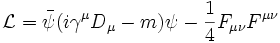.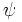and its Dirac adjoint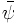are the fields representing electrically charged particles, specifically electron and positron fields represented as Dirac spinors.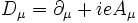is the gauge covariant derivative, with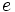the coupling strength (equal to the elementary charge),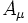the covariant vector potential of the electromagnetic field and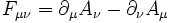the electromagnetic field tensor. Also, γμ are Dirac matrices. The part of the Lagrangian containing the electromagnetic field tensor describes the free evolution of the electromagnetic field, whereas the Dirac-like equation with the gauge covariant derivative describes the free evolution of the electron and positron fields as well as their interaction with the electromagnetic field.The one-loop contribution to the vacuum polarization function ΠThe one-loop contribution to the electron self-energy function Σ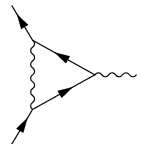The one-loop contribution to the vertex function Γ See also Quantum field theory Basics of quantum mechanics Gauge theory quantum gauge theory Gupta-Bleuler formalism vacuum polarization vertex function anomalous magnetic moment self-energy Renormalization Landau pole quantum chromodynamics Schwinger model Standard Model positronium Lamb shift Compton scattering Bhabha scattering Moeller scattering Cavity quantum electrodynamics (Cavity QED) References R. P. Feynman, 1985. QED: The strange theory of light and matter [ISBN 0691024170] Claude Cohen-Tannoudji, Jacques Dupont-Roc, Gilbert Grynberg, Photons and Atoms : Introduction to Quantum Electrodynamics (John Wiley & Sons, 1997) [ISBN 0471184330] J. M. Jauch, F. Rohrlich, The Theory of Photons and Electrons (Springer-Verlag, 1980) R. P. Feynman, Quantum Electrodynamics (Perseus Publishing, 1998) [ISBN 0201360756] Schweber, Silvian S. ; Q.E.D. and the men who made it: Dyson, Feynman, Schwinger, and Tomonaga, Princeton University Press (1994) [ISBN 0-691-03327-7] Miller, Arthur I. ; Early Quantum Electrodynamics : A Sourcebook, Cambridge University Press (1995) [ISBN 0521568919] Schwinger, Julian ; Selected Papers on Quantum Electrodynamics, Dover Publications, Inc. (1958) [ISBN 0-486-60444-6] Hiçbir yazı/ resim  izinsiz olarak kullanılamaz!!  Telif hakları uyarınca bu bir suçtur..! Tüm hakları Çetin BAL' a aittir. Kaynak gösterilmek şartıyla  siteden alıntı yapılabilir.  © 1998 Cetin BAL - GSM:+90  05366063183 -Turkiye/Denizli  [ Ana Sayfa (Home) |  UFO Technology |  UFO's Galleri | Kuantum Fiziği | Roket bilimi   |                                              [ Astronomy |  E-Mail   |  Index |